Traveltime approximations for transversely isotropic media with an inhomogeneous backgroundNext: The vertical direction Up: Alkhalifah: TI traveltimes in Previous: Expansion in terms of

# A homogeneous model test

Though the equations above are developed for a general inhomogeneous background medium, I examine their accuracy in representing TI traveltime and traveltime moveout in the homogeneous case. This is convenient since most parameter scan-type applications (i.e. semblance velocity analysis) are performed considering an effective homogeneous medium.

As shown in Appendix C, I use the simple traveltime relation for an elliptically anisotropic homogeneous background to recursively solve for the coefficients of the traveltime expansion inand, and thus, obtain analytical representations for coefficients,,,,, and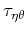. Setting, to allow for a simplified presentation, I obtain an analytical representation of traveltime for tilted elliptical anisotropy given by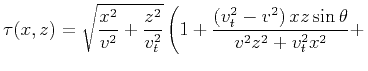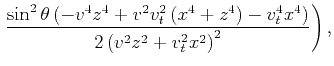(9)

where the source is located atand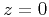.

This formula type (equation 9) basically represents a moveout equation for traveltime in TI media as a function of offset (or) and can be compared with equations developed explicitly to represent the moveout in TI media. Pech et al. (2003) derived the exact quartic moveout coefficient (i.e., the fourth-order term of the Taylor series expansion for squared traveltime) for pure (nonconverted) reflections in arbitrarily anisotropic, heterogeneous media. They also linearized the P-wave quartic coefficient in the anisotropy parameters for homogeneous tilted TI media above a horizontal and dipping reflector. For a horizontal TI layer, the P-wave fourth-order Taylor series with linearized expressions for both the NMO velocity (Grechka and Tsvankin, 2000) and the quartic coefficient (Pech et al., 2003) is given by:(10)

where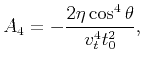(11)is the two-way zero-offset time andis the offset (). For zero tilt, it reduces to(12)

which reveals the additional approximation involved in this equation as even the second-order term is linearized with respect to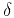. Meanwhile, settingin equation 9 yields an accurate description of the second-order term of the moveout with the NMO velocity in the denominator instead.

Grechka and Pech (2006) suggested rewriting equation 10 in the following form:(13)

which typically provides higher-order accuracy as the additional offset component in the denominator of the fourth-order term tends to predict the behavior at very large offsets.

Sena (1991) presented an anisotropic approximation of the group velocity as a function of symmetry angle. These velocities can serve to obtain moveout equations as well, and they are given by(14)

where the ray angle,, satisfies(15)

Using an elliptical anisotropic background model with axis-direction velocity equal to 2 km/s velocity,, and tilt angle=, I compare the traveltime errors of the moveout equations extracted from our eikonal based formulations with those that are used for pure moveout approximations, equations 13 and 14. For a reflector at depth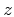=2 km, Figure 2 shows the percentage traveltime errors as a function of offset for the equations given above. Clearly, equations 13 and 14, given by the solid grey and dashed curves, respectively, are less accurate in describing the traveltime behavior overall than the new formula (solid black curve), equation 9. The moveout equations have faired well for the vertical direction but performed poorly for larger offset.errTEll3f
Figure 2.
The relative traveltime error as a function of offset for an elliptically anisotropic model with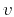=2 km/s,,, and a reflector depth=2 km for the new expansion in(solid black curve), the nonhyperbolic moveout equation 10 (solid gray curve), and for the Sena approximation (dashed black curve).Moreover, for a more practical case of TI, in which=0.2, the errors, as shown in Figure 3, are smaller overall for the new equation (solid black curve) than the other approximations. Despite the larger error of our approximation near vertical, the departure of the other approximations from the accurate value at large offset reflect their near-offset based expansion. In the new equation, the expansion is with respect to small tilt, and thus, has an unbiased representation of the traveltime with respect to offset. As a result, the accuracy of the new equation is higher over all.errTTI3fNew
Figure 3.
The relative traveltime error as a function of offset for a model with=2 km/s,=0.2,, and a reflector depth=2 km for the new expansion in(solid black curve), the nonhyperbolic moveout equation 10 (dashed gray curve), and 13 (solid gray curve) and for Sena's approximation (dashed black curve).The analytical equations developed in this section were meant to test the accuracy of the perturbation theory approximations applied to the eikonal equation. Though they show high accuracy in representing the moveout, the general perturbation formulations are not meant to be only used as an alternative to other available equations derived for the homogeneous case. Their perturbation from a background inhomogeneous model allows us to predict traveltime in more complex media.Traveltime approximations for transversely isotropic media with an inhomogeneous backgroundNext: The vertical direction Up: Alkhalifah: TI traveltimes in Previous: Expansion in terms of

2013-04-02